25 POINTS AND BRAINIEST BADGE NO LINKS NO PICTURE FOR QUESTION A parallelogram has vertices at (-5, -1), (-2, -1), (

Question

NO PICTURE FOR QUESTION

A parallelogram has vertices at (-5, -1), (-2, -1), (-3, -4), and (-6, -4). What is the approximate perimeter of the parallelogram? Round your answers to the nearest hundredth.
A. 9.16
B. 12 units
C. 12.32 units
D. 14

in progress 0
6 months 2021-07-17T08:58:50+00:00 2 Answers 0 views 0

1. It is A because it can’t be B and it can’t be C and it can’t be D so my answer is A

12.32 units

Step-by-step explanation:

Use the distance formula to find the lengths between two points: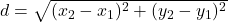the distance between (-2, -1) and (-5,-1) is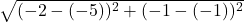=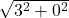=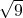=3

the distance between (-2, -1) and (-3, -4) is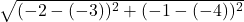=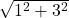=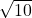≈3.16

the distance between (-6, -4) and (-3, -4) is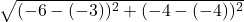=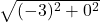==3

the distance between (-6, -4) and (-5, -1) is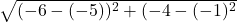=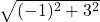=≈3.16